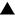Algebra 1 section of MathBitsNotebook.comAlgebraic ExpressionsThis section on Algebraic Expressions will be a "refresher" of many familiar concepts.
In these lessons, familiar concepts will be restated and then further developed.indicates compatibility to NY Next Generation Math Standards (NGMS)
Algebraic Expressions (definition, parts, classifications by term, by degree)Combining Like Terms (like terms, combining, simplifying expressions)Translating to Algebraic Expressions (translate words to algebraic notation)Factoring Linear Expressions (binomial, GCF, Distributive Property in reverse)Consecutive Integer ExpressionsPRACTICE: • Algebraic Expressions (format, translating, applications)• Manipulating Algebraic Expressions (combine, evaluate, equivalent, factor)• Consecutive Integer Expressions Practice